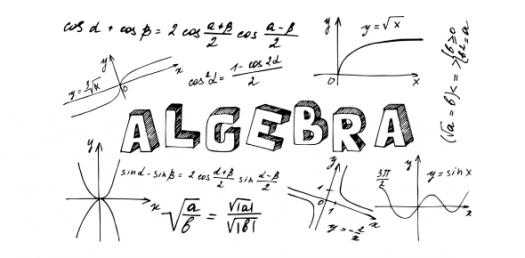# Algebra 2: Sect. 1.1 - 1.2 Test

Approved & Edited by ProProfs Editorial Team
The editorial team at ProProfs Quizzes consists of a select group of subject experts, trivia writers, and quiz masters who have authored over 10,000 quizzes taken by more than 100 million users. This team includes our in-house seasoned quiz moderators and subject matter experts. Our editorial experts, spread across the world, are rigorously trained using our comprehensive guidelines to ensure that you receive the highest quality quizzes.
| By Gdyson
G
Gdyson
Community Contributor
Quizzes Created: 17 | Total Attempts: 19,200
Questions: 15 | Attempts: 396SettingsTest includes linear functions as tables, equations, and graphs. Also slope and intercept by graphing and formula.

• 1.

### Question A Which of these points does not lie on the graph of y = -3x - 4? A)  (0 , -4) B)  (1 , -1) C)  (-1 , -1) D)  (-2 , 2)

• A.

A

• B.

B

• C.

C

• D.

D

B. B
Explanation
The point (1, -1) does not lie on the graph of y = -3x - 4 because when we substitute x = 1 into the equation, we get y = -3(1) - 4 = -3 - 4 = -7, not -1.

Rate this question:

• 2.

• A.

A

• B.

B

• C.

C

• D.

D

B. B
• 3.

### Question C A family membership at a swimming pool costs a flat fee of \$100, plus \$50 per person.  If the letter n stands for the  number of people, and C is the total cost, then the equation that best describes this situation is:   A) C = 50n + 100  B) C = 100n + 50 C) C = 100 + 50 + n D) C = 150n

• A.

A

• B.

B

• C.

C

• D.

D

A. A
Explanation
The equation that best describes the situation is C = 50n + 100. This is because there is a flat fee of \$100, which is added to the cost of \$50 per person (n). Therefore, the total cost (C) is calculated by multiplying the number of people (n) by \$50 and adding the flat fee of \$100.

Rate this question:

• 4.

• A.

A

• B.

B

• C.

C

• D.

D

C. C
• 5.

• A.

A

• B.

B

• C.

C

• D.

D

B. B
• 6.

• A.

A

• B.

B

• C.

C

• D.

D

C. C
• 7.

• A.

A

• B.

B

• C.

C

• D.

D

B. B
• 8.

• A.

A

• B.

B

• C.

C

• D.

D

A. A
• 9.

• A.

A

• B.

B

• C.

C

• D.

D

D. D
• 10.

• A.

A

• B.

B

• C.

C

• D.

D

D. D
• 11.

• A.

A

• B.

B

• C.

C

• D.

D

D. D
• 12.

### Question M Using the equation y = -4x - 2,  what will be the y-coordinate of a point whose x coordinate is  5?

• A.

-11

• B.

18

• C.

-22

• D.

12

C. -22
Explanation
The equation y = -4x - 2 represents a linear function in slope-intercept form, where the coefficient of x is the slope and the constant term is the y-intercept. In this case, the slope is -4 and the y-intercept is -2. To find the y-coordinate of a point with x = 5, we substitute x = 5 into the equation: y = -4(5) - 2 = -20 - 2 = -22. Therefore, the y-coordinate of the point is -22.

Rate this question:

• 13.

### Question N                Using the graph below, determine the slope of this line:

• A.

-3/6

• B.

3/5

• C.

-2/6

• D.

2/5

D. 2/5
Explanation
The slope of a line is determined by the ratio of the change in the y-coordinates to the change in the x-coordinates between any two points on the line. In this case, the line passes through the points (0, -3) and (5, 3). The change in the y-coordinates is 3 - (-3) = 6, and the change in the x-coordinates is 5 - 0 = 5. Therefore, the slope of the line is 6/5, which is equivalent to 2/5.

Rate this question:

• 14.

### Question O What is the equation of the line below in y = mx + b form?

• A.

Y = -2x

• B.

Y= -1/2 x + 1

• C.

Y = -1/2 x + 2

• D.

Y = 1/2 x + 1

B. Y= -1/2 x + 1
Explanation
The equation of the line in y = mx + b form is y = -1/2x + 1 because it follows the standard form of a linear equation. The coefficient of x, -1/2, represents the slope of the line, which indicates that the line is decreasing as x increases. The constant term, 1, represents the y-intercept, which is the point where the line crosses the y-axis. Therefore, the equation y = -1/2x + 1 accurately represents the given line.

Rate this question:

• 15.

### Question P Change the equation  -3x + 5y = 10 into slope-intercept form.

• A.

5y = 3x + 10

• B.

Y = 3x + 10

• C.

Y = 3/5 x + 2

• D.

Y = 5/3 x + 10/3